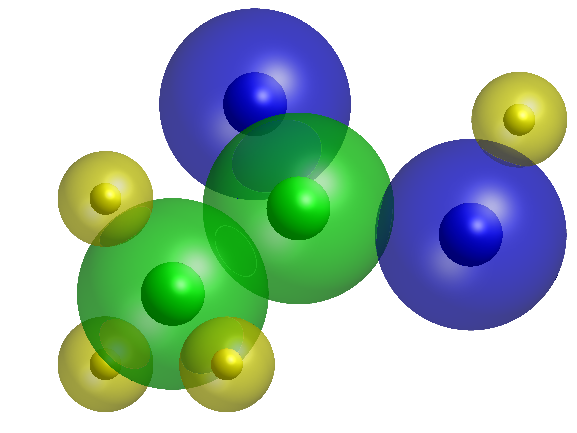# Acetic Acid MoleculeEric Ludlam on 27 Oct 2021
• 3
• 19
• 0
• 0
• 276
% Acetic Acid
%
% BLUE = oxygen
% GREEN = carbon
% YELLOW = hydrogen
% Run pdb file thru a character encoder, paste in here, and decode.
% Reduces overall size of the data by hudreds of char.
% Note: Encoder offsets by some amount into printable character range, but no
% need to undo that here due to auto limit scaling.
C='242(g2B2(b?-2(b%,2(gD0;\$y /)\$y /;\$y''"2\$y';
D=double(C)/10-3.2;
[X,Y,Z]=sphere(99);
% Draw 2 nested surfaces in as compact a way as I can find.
r=@(i,s,a)surface(s*X+D(i),s*Y+D(i+1),s*Z+D(i+2),FaceA=a);
a=@(i)set([r(i,D(i+3),.5)
r(i,D(i+3)/3,1)],FaceC=C(i+4),EdgeC='n');
for q=1:5:40
a(q);
end
view([-15 40])
axis equal off
lighting g
camlight
camva(5)